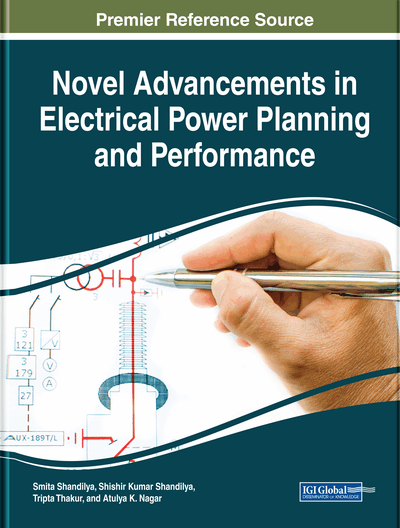# Application of Moth-Flame Optimization Algorithm for the Determination of Maximum Loading Limit of Power System: Application of MFO for Maximum Loading Limit

Suvabrata Mukherjee (NSHM Durgapur, India) and Provas Kumar Roy (Kalyani Government Engineering College, India)
Copyright: © 2020 |Pages: 16
DOI: 10.4018/978-1-5225-8551-0.ch003
OnDemand PDF Download:
Available
\$37.50
No Current Special Offers

## Abstract

Moth-flame optimization algorithm (MFOA) based on the navigation strategy of moths in universe is a novel bio-inspired optimization technique and has been exerted for determining the maximum loading limit of power system. This process is highly effective for traversing long distances following a straight path. As a matter of fact, moths follow a deadly spiral path as artificial lights tend to confuse them. Exploration and exploitation are two vital aspects of the algorithm, used in tuning of the parameters. The algorithm is verified on MATPOWER case30 and case118 systems. Comparison of the performance of MFOA has been done with other evolutionary algorithms such as multi-agent hybrid PSO (MAHPSO), differential evolution (DA), hybridized DE, and PSO (DEPSO). The performance of MFOA in determining maximum loading limit is verified from the results. In much reduced time, MFO algorithm also gives high maximum loading point (MLP).
Chapter Preview
Top

## 1. Introduction

Being considered as the biggest man-made gadget in the world, electric power grid comprises of transmission lines transformers, synchronous generators, active and reactive components, switches, relays, and loads. Being nonlinear and complex in nature, power systems are prone to faults and disturbances. Increased loading causes heavy stress on the power systems that may cause voltage instability problems. Numerous studies in recent times are being carried out for detection of loading limits of power systems related to security monitoring and voltage stability. Maximum loading limit (MLL) can be defined as the periphery between the maximum loading point (MLP) and operating point of the system (Ajjarapu and Christy, 1992; Irisarri et al., 1997).Various control parameters operation in such a system requires providing a solution to an optimization problem (Sato, 2002; Karysinos et al, 2005).Among some of the methods; the continuation power flow (CPF) is a widely used approach. However, with increase in step length, the method is inefficient in providing an accurate result. Another effective approach used for solving the maximum loading problem is the Interior point method (IP). However, there are limitations in initial and concluding conditions. In sequential quadratic programming (SQP), differentiation of the constraints is an important aspect. But calculation of large number of matrices during the iteration process makes the method complex and sluggish. An efficient approach that has been used for determination of maximum loading limit is the Fuzzy logic technique (Babulal et al., 2008). However, the results highlight its inefficiency to provide global optima.

Recently, many researchers have used evolutionary algorithms for determining the MLL. Not heavily afflicted by nonlinearity of the problem, the Particle swarm optimization (PSO) is an efficient computational intelligence-based approach. Because of these advantages, for most problems, PSO can converge to the optimal solution (del Valle et al, 2008). By fusing breeding of genetic algorithm, the performance of PSO is enhanced (EL-Dib Amgadet al, 2006) which has been again used for determining the MLL (EL-Dib Amgadet al, 2006). The article, however, has not considered the voltage limit. Also the hybrid optimization technique is unsuitable for large systems. Determining the optimum generator allocation at the MLP, MLL has been determined using the Multi agent hybrid PSO (MAHPSO) (Shunmugalatha et al., 2008) where till the voltage limits are breached, the load is increased uniformly in all the load buses. MLL is also determined using co-ordinated aggregation based particle swarm optimization (CAPSO) (Arya et al, 2010) and genetic algorithm (GA) (Acharjee, 2012). In this case the voltage stability limit where the voltage magnitude is much lower than the lower limit is assumed to be the maximum limit. MLL is also examined considering the dynamic security and steady state constraints (Gan et al., 2003).

A very successful evolutionary computation algorithm is the differential evolution algorithm (DEA).DEA has excellent convergence characteristics and is easy to understand and implement because of the fewer number of control parameters to be provided by the users (Shunmugalatha et al., 2008; Acharjee, 2012; Arya et al., 2010). Although DEA is studied to be fast, robust and precise, the faster convergence property sometimes causes premature convergence. Also there is a high probability in searching tendency in the direction of local optima.

## Complete Chapter List

Search this Book:
Reset